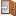### Introduction to theory of elementary particles 2017Content of the lecture:

Classification of particles, intuition, kinematics, etc.

S-matrix and cross section formula, QED Feynman rules

Discrete symmetries

Lie groups and representation theory

Quark model, particles spectrum

Parton model

QCD

Goldstone Theorem

Chiral Symmetry

Gauge theory and spontaneous symmetry breaking

Fermi model

$SU(2)\times U(1)$ symmetry

Elements of the standard model

Neutrino oscillations